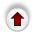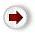## 3.1. Balanced Trees

We have seen that the efficiency of many important operations on trees is related to the Height of the tree - for example searching, inserting, and deleting in a BST are all O(Height).

In general, the relation between Height (H) and the number of nodes (N) in a tree can vary from H = N (degenerate tree) to H = log(N).

For efficiency's sake, we would like to guarantee that H was O(logN). One way to do this is to force our trees to be height-balanced.A tree is perfectly height-balanced if the left and right subtrees of any node are the same height. e.g.

`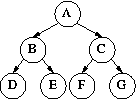`
It is clear that at every level there are twice as many nodes as at the previous level, so we do indeed get H = O(logN). However, perfect height balance is very rare: it is only possible if there are exactly 2^H-1 nodes!

As a practical alternative, we use trees that are `almost' perfectly height balanced. We will say that a tree is height-balanced if the heights of the left and right subtree's of each node are within 1. The following tree fits this definition:

``
We will say this tree is height-balanced.

How can we tell if a tree is height-balanced? We have to check every node. The fastest is way is to start at the leaves and work your way up. When you reach a node, you will know the heights of its two subtrees; from that you can tell whether it is height-balanced and also compute the node's height (for use by its parent). For example, in the tree above

`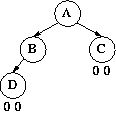`
C and D are leaves. Their subtrees are all height 0 so C and D are both perfectly balanced. Having finished D we can compute the heights of B's subtrees.
``
B is not perfectly balanced, but the heights of of its subtrees differ only by 1, so B is regarded as height-balanced. Now we can compute the balance of A.
`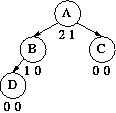`
Like B, A's two subtrees differ by 1 in height. We have now looked at every node; every one is height-balanced, so the tree as a whole is considered to be height-balanced.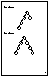`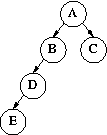`
``